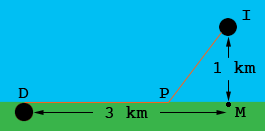Julie Grade 12 Parent of Student Mrs. Faria lives on an island 1 km from the mainland. She paddles her canoe at 3 km/h and jogs at 5 km/h. the nearest drug store is 3 km along the shore from the point on the shore closest to the island. Where should she land to reach the drug store in minimum time? Hi Julie, I drew a diagram and labeled some points.I is the island, D is the drug store, M is the point on the mainland closest to the island and P is the point where Mrs. Faria' canoe lands, so she paddles from I to P and then jogs from P to D. Let x be the distance from M to P in km. Use Pythagoras Theorem to find an expression for the distance from I to P. Now, since distance is timerate you can find an expression for the time it takes her to paddle from I to P. Since the distance from P to M is x km, the distance from P to D is 3 - x km. Again distance is timesrate so you can find the time it takes her to jog from P to D. Add the two times to get a total time to go from I to D and then use calculus to find the value of x that minimize this time. Penny